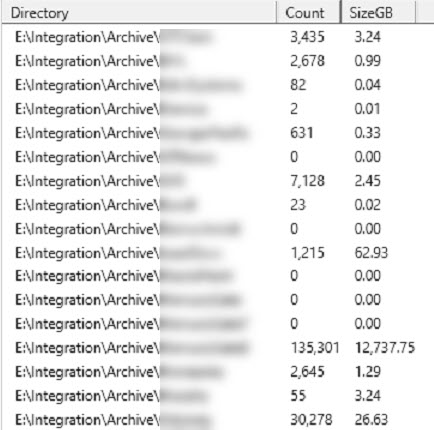## Powershell to get total disk space used per directory

With BizTalk, we often archive files in a disk directory, where each Vendor has its own archive.

#### Simple Example

I found the simple example below, then expanded upon:

``````<pre>
Get-ChildItem E:\Archive | Measure-Object -Property Length -sum
</pre>``````

#### Fancy Example

This starts with the Archive directory then basically runs the command above for each subdirectory. One trick was to get the variables from the “measure-object”, and the second trick was how to present the data. Just using Write-Host statements looked very ugly.

To present the data, I wanted to use the Out-GridView. To do that, I had to load a HashTable in a list, and pass that List to the Out-GridView. I haven’t got all the numbers right-justified yet, perhaps soon.

``````<pre>
\$topDirectories = Get-ChildItem \$startDir -Directory
\$countDir = 0
\$List = New-Object System.Collections.ArrayList

foreach (\$dir in \$topDirectories)
{
\$countDir = \$countDir + 1
#if (\$countDir -gt 1) {exit}
#Write-Host "Dir: \$(\$dir.FullName)"
\$measure = Get-ChildItem \$dir.FullName -Recurse | Measure-Object -Property Length -sum
Write-Host \$dir.BaseName \$measure.Count \$measure.Sum

## Empty directory seems to have null values
if (\$measure.Sum -eq -\$null)
{
\$SizeMB = 0
}
else
{
\$SizeMB = \$measure.Sum / 1024 / 1024
}

\$Hash = [ordered]@{
Directory=  \$dir.Fullname
Count = \$measure.Count
SizeMB = [math]::Round(\$SizeMB *100) / 100   #round to two decimal places
}